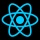Home
IT Knowledge
Inspiration
Languages
EN

# Python - math.asin() method example

0 points
Created by:Admin Dirask Community
1270

The `math.asin` function returns number in radians in the range `-math.pi/2` to `+math.pi/2`. The function calculates the inverted sine function value.

``````import math

def calculateAngle(b, h):
return math.asin(b / h)

#   |\
#   | \ h
# a |  \
#   |__*\ <- angle
#     b

# a, b and h build isosceles right triangle
a = 3
b = a
h = math.sqrt(a * a + b * b)
print(calculateAngle(b, h))  # 0.7853981633974483 <-  45 degrees

# a, b and h build half of equilateral triangle
a = 3
b = a * math.sqrt(3)
h = math.sqrt(a * a + b * b)
print(calculateAngle(b, h))  # 0.5235987755982987 <- ~30 degrees

# a, b and h are not able to build triangle
a = 3
b = a
h = 1
print(calculateAngle(b, h))  # ValueError``````

## 1. Documentation

 Syntax ``math.asin(number)`` Parameters `number` - double value that represents the result of the operation `adjacent / hypotenuse` on the right triangle. `number` should be in the range `-1` to `+1`. Result `number` value in radians in the range `-math.pi/2` to `+math.pi/2`. If the value can not be calculated `ValueError` occurs. Description `asin` is a method that takes only one parameter and returns an approximation of the result of the mathematical function arcsine(x).

## 2. Working with degrees

``````import math

def calculateAngle(b, h):
return math.asin(b / h)

#   |\
#   | \ h
# a |  \
#   |__*\ <- angle
#     b

# a, b and h build isosceles right triangle
a = 3
b = a
h = math.sqrt(a * a + b * b)
print(calculateAngle(b, h))  # 0.7853981633974483 <-  45 degrees

# a, b and h build half of equilateral triangle
a = 3
b = a * math.sqrt(3)
h = math.sqrt(a * a + b * b)
print(calculateAngle(b, h))  # 0.5235987755982987 <- ~30 degrees

# a, b and h are not able to build triangle
a = 3
b = a
h = 1
print(calculateAngle(b, h))  # ValueError``````

## References

1. Inverse trigonometric functions - Wikipedia

## Python - math module

##### 🚀
Get your tech brand or product in front of software developers.
For more information Contact us

Join